# 第 8 章 马尔科夫链蒙特卡洛抽样

Monte Carlo方法，通常可追溯到1940年代后期的冯诺依曼在曼哈顿计划中的应用。第一个MCMC算法与 另一台名为MANIAC的计算机相关，该计算机是在1952年初在Metropolis的指挥下在洛斯阿拉莫斯实验室 建造。以当时项目参与人物理学家和数学家Metropolis命名的Metropolis算法一直被使用到如今。

Metropolis算法后来Hastings在1970年Metropolis算法后来被推广和扩展，形成了Metroplis-Hastings 算法。Hastings和他的学生Peskun于1973年，1981年把该算法推广作为作为一般的统计模拟工具。

Gelfand和Smith在1990年的一篇论文大量使用了MCMC方法，之后激发了新的贝叶斯方法，统计计算中使 用Gibbs抽样和Metropolis-Hastings算法。有趣的是，Tanner和Wong（1987）的论文与Gelfand和Smith （1990）的论文，证明从条件分布模拟就足够模拟联合分布渐近分布。这在理论上证明了MCMC方法的科 学性。至此以后，MCMC方法得到了蓬勃发展，已经成为机器学习，统计计算中最为核心的计算工具之一。

## 8.1 介绍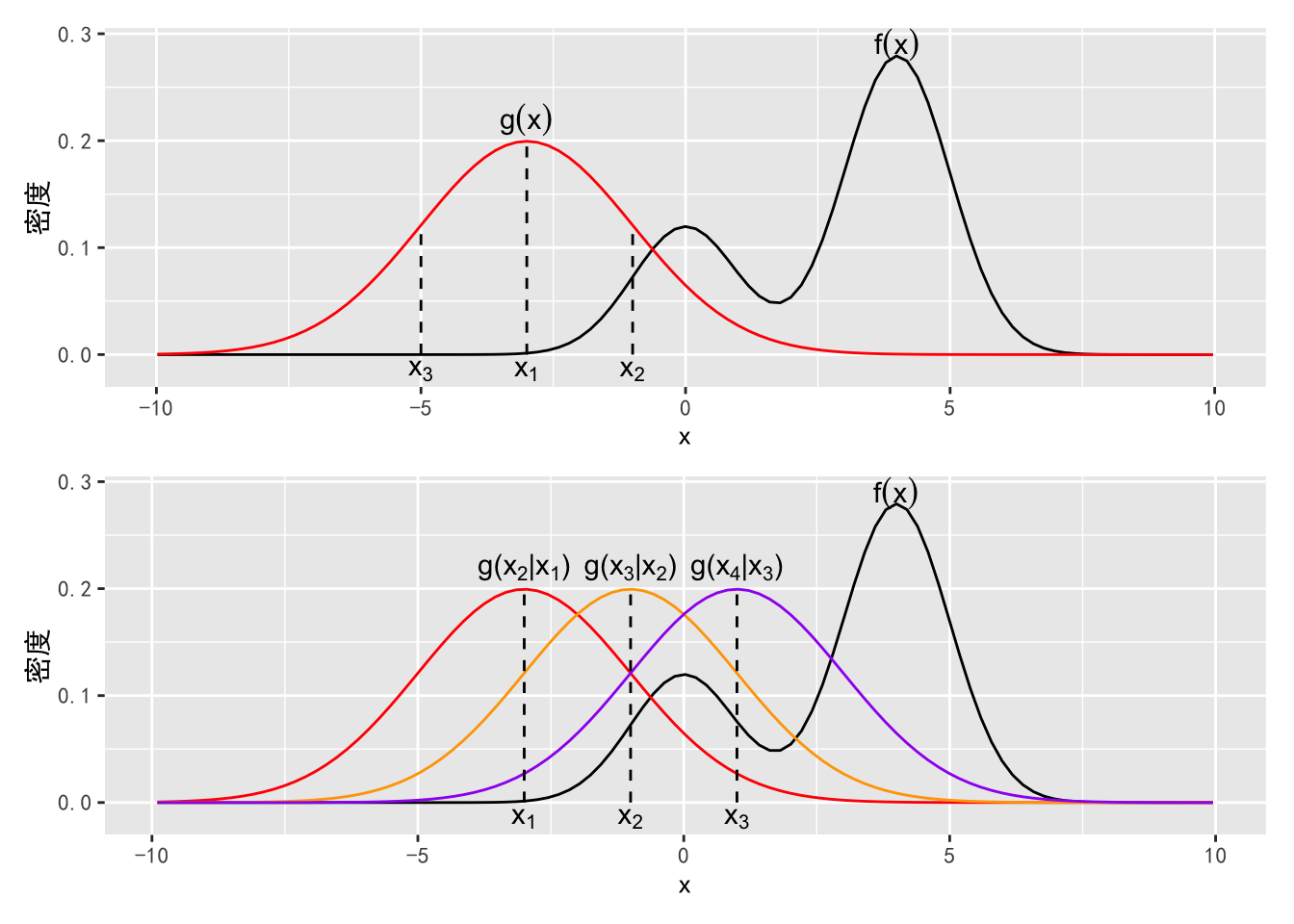## 8.3 Metropolis-Hastings

Metropolis-Hastings 算法

1. $$g(x^*|x_{t-1})$$ 中抽取随机数 $$x^*$$

2. 计算接受概率

$\alpha = \min\left(1, \frac{f(x^*)g(x_{t-1}|x^*)}{f(x_{t-1)}g(x^*|x_{t-1}))}\right).$

1. 产生均匀分布的随机数 $$U \sim U(0,1)$$，如果 $$U \leq \alpha$$，接受 $$x^*$$，即以 $$\alpha$$ 为接受概率接受 $$x^*$$。若接受，$$x_t = x^*$$，否则 $$x_t = x_{t-1}$$

### 8.3.1 随机游走 Metropolis-Hastings

• $$q$$ 中抽取 $$\epsilon$$，其中 $$q$$ 是一个以 0 为中心的对称分布。让 $$x^* = x_{t-1} + \epsilon$$

• 计算接受概率

$\alpha = \min\left(1, \frac{f(x^*)}{f(x_{t-1})}\right).$

• 产生均匀分布的随机数 $$U \sim U(0,1)$$，如果 $$U \leq \alpha$$，接受 $$x^*$$，即以 $$\alpha$$ 为接受概率接受 $$x^*$$。若接受，$$x_t = x^*$$，否则 $$x_t = x_{t-1}$$

f <- function(x) {
0.3 * dnorm(x, 0, 1) + 0.7 * dnorm(x, 4, 1)
}
ggplot(data.frame(x=runif(1000, -5, 8)), aes(x)) +
stat_function(fun = f) +
ylab('f(x)')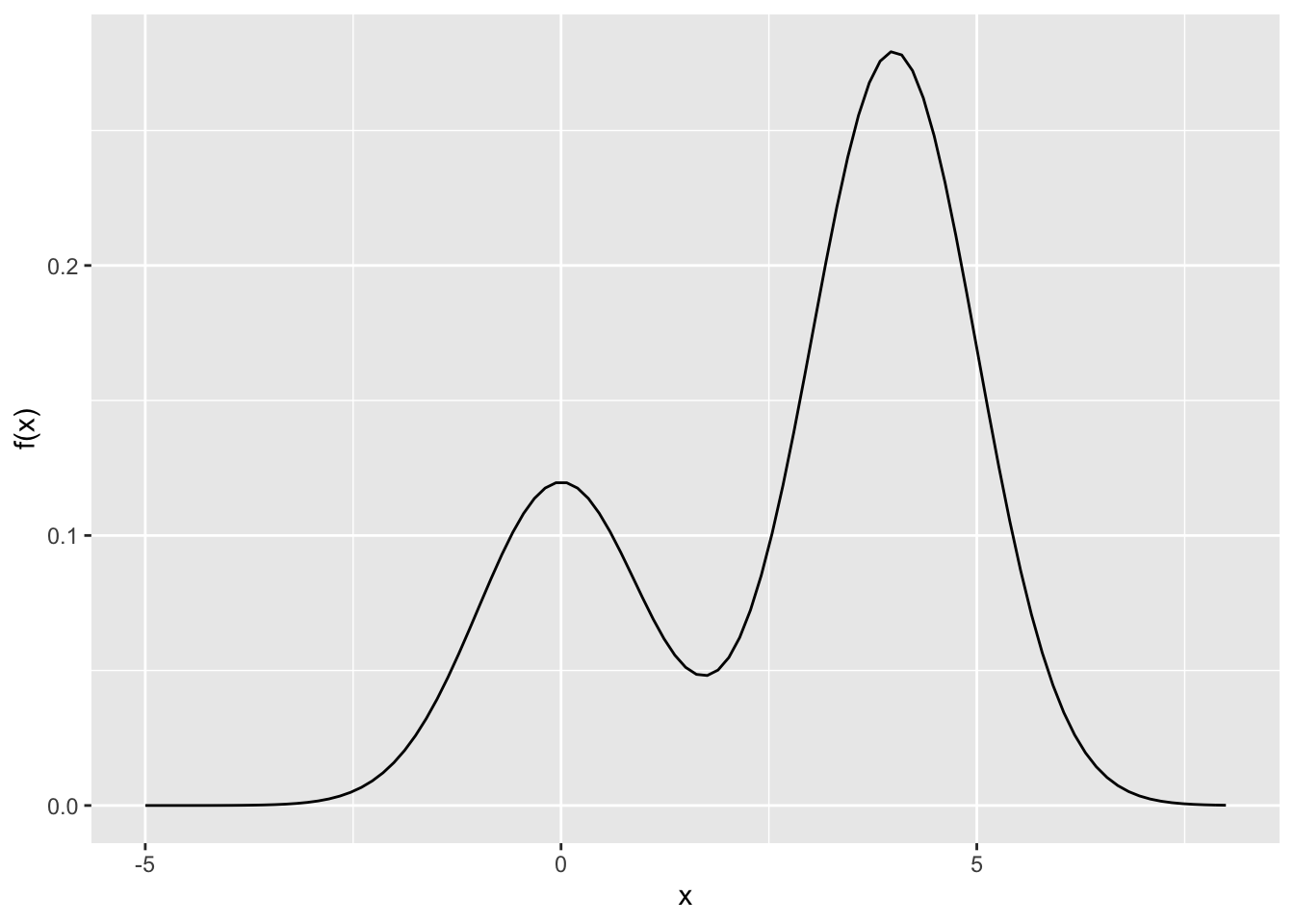g <- function(x) rnorm(1, x, 4)

rw.mh <- function(x0, f, g, n.steps = 1000, ...){
x <- x0
samples <- matrix(NA, n.steps, length(x))
for (i in seq_len(n.steps)){
# 猜想
x.new <- g(x)
# 计算接受概率
alpha <- min(1, f(x.new, ...) / f(x, ...))
# 判断是否接受
if (runif(1) < alpha){
x <- x.new
}
samples[i,] <- x
}
return(samples)
}

x0 <- 1
n.steps <- 2000
samples <- rw.mh(x0, f, g, n.steps = n.steps)

hist(samples, prob =TRUE)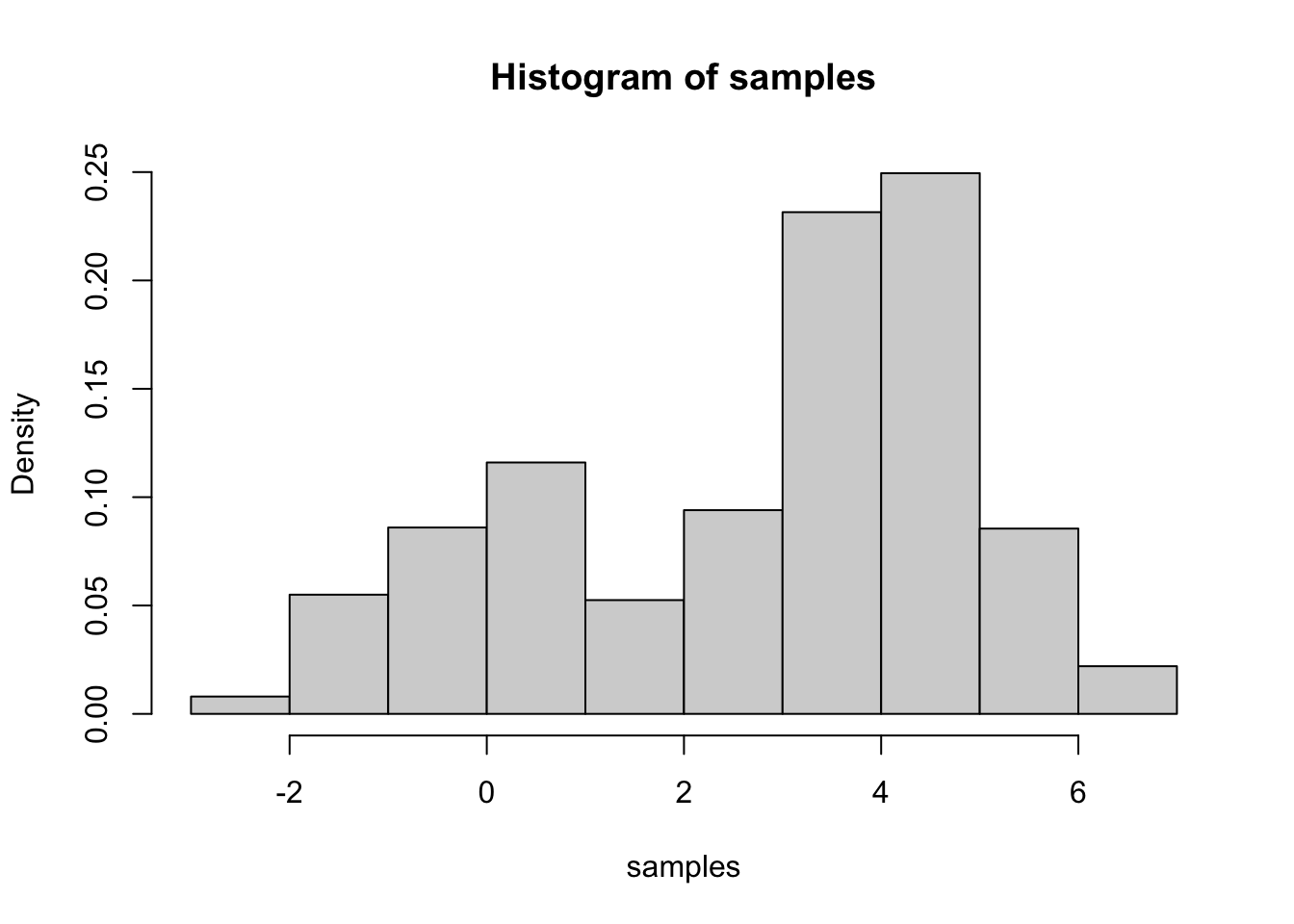qplot(1:n.steps, samples, geom = "line", xlab = "迭代次数",
ylab = "抽样") +
theme(text = element_text(family = "STSong"))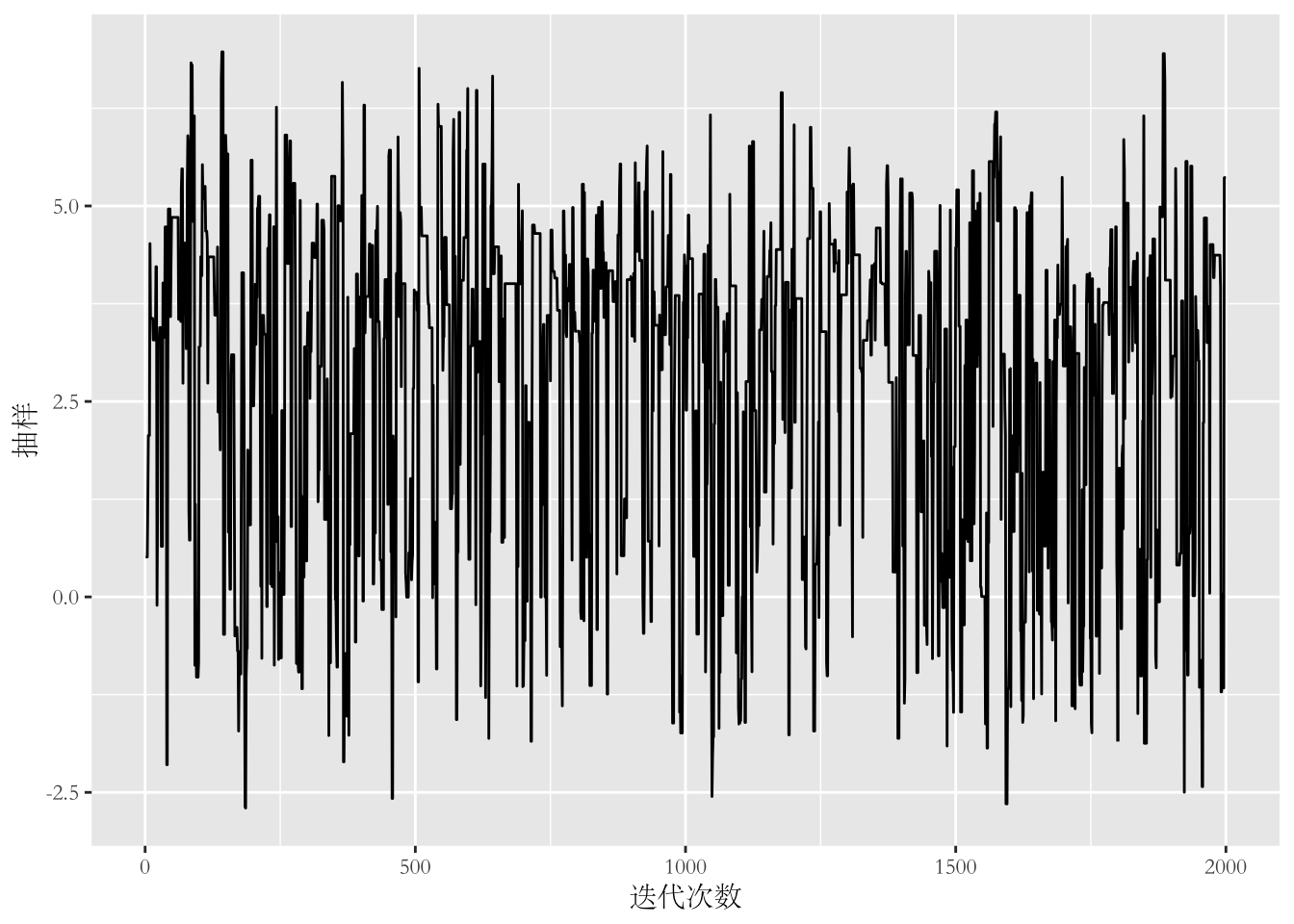f <- function(x,
mu = c(-1, 1),
Sigma = matrix(c(1, .25, .25, 1.5), 2, 2)){
logdet <- as.numeric(determinant(Sigma, TRUE)\$modulus)
cons <- length(mu) * log(2 * pi) + logdet
Sigma.i <- solve(Sigma)
dx <- x - mu
exp(-(cons + rowSums((dx %*% Sigma.i) * dx))/2)
}
x <- seq(-4, 1.5, length = 100)
y <- seq(-2, 4, length = 100)
xy <- expand.grid(x = x, y = y)
z <- matrix(apply(as.matrix(xy), 1, f), length(x), length(y))
image(x, y, z, las = 1)
contour(x, y, z, add = TRUE)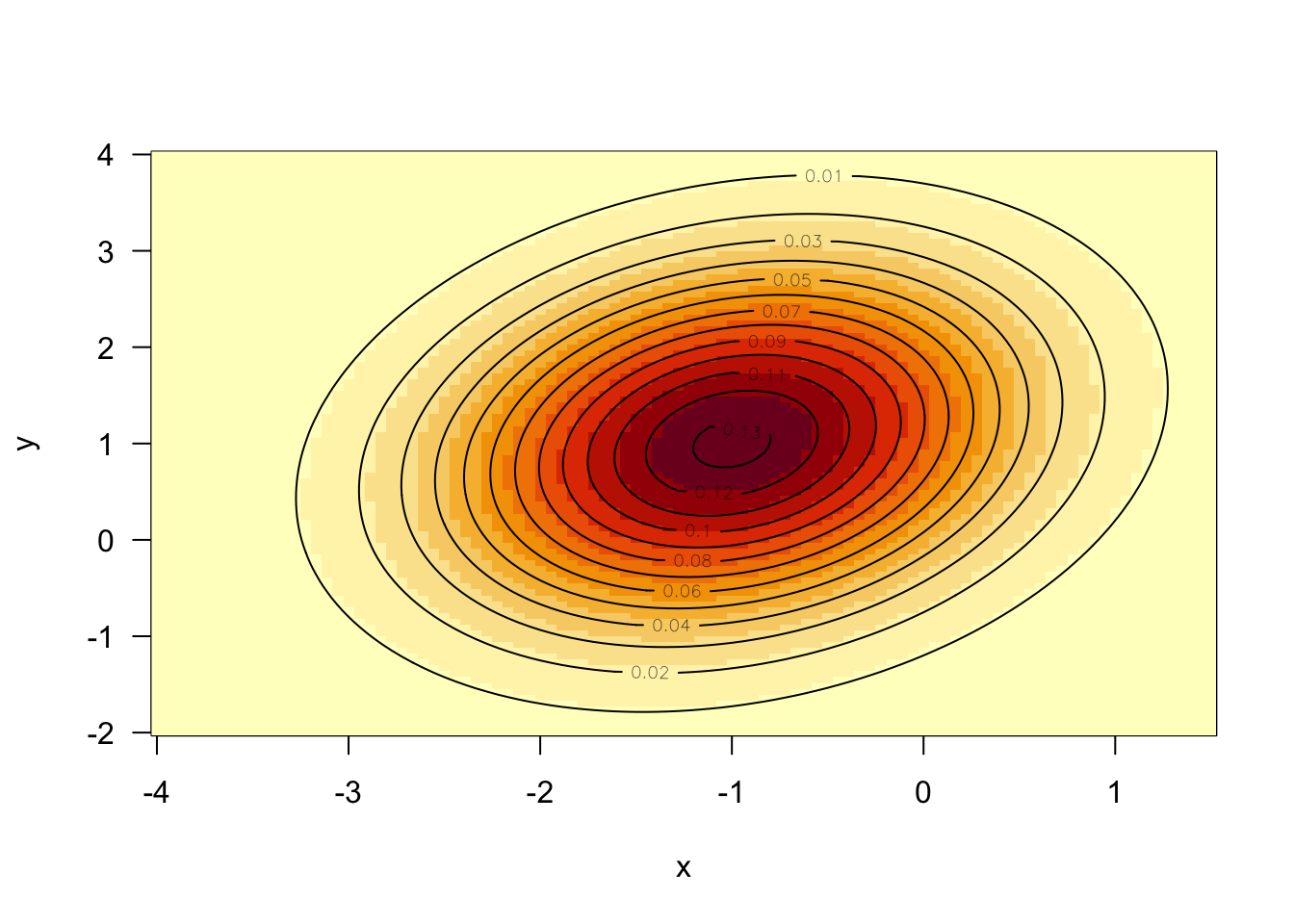g <- function(x, d = 2){
x + runif(length(x), -d/2, d/2)
}

set.seed(Sys.Date())
samples <- rw.mh(c(-4, 4), f, g, 10000)
smoothScatter(samples, xlab ='x', ylab = 'y')
contour(x, y, z, add = TRUE)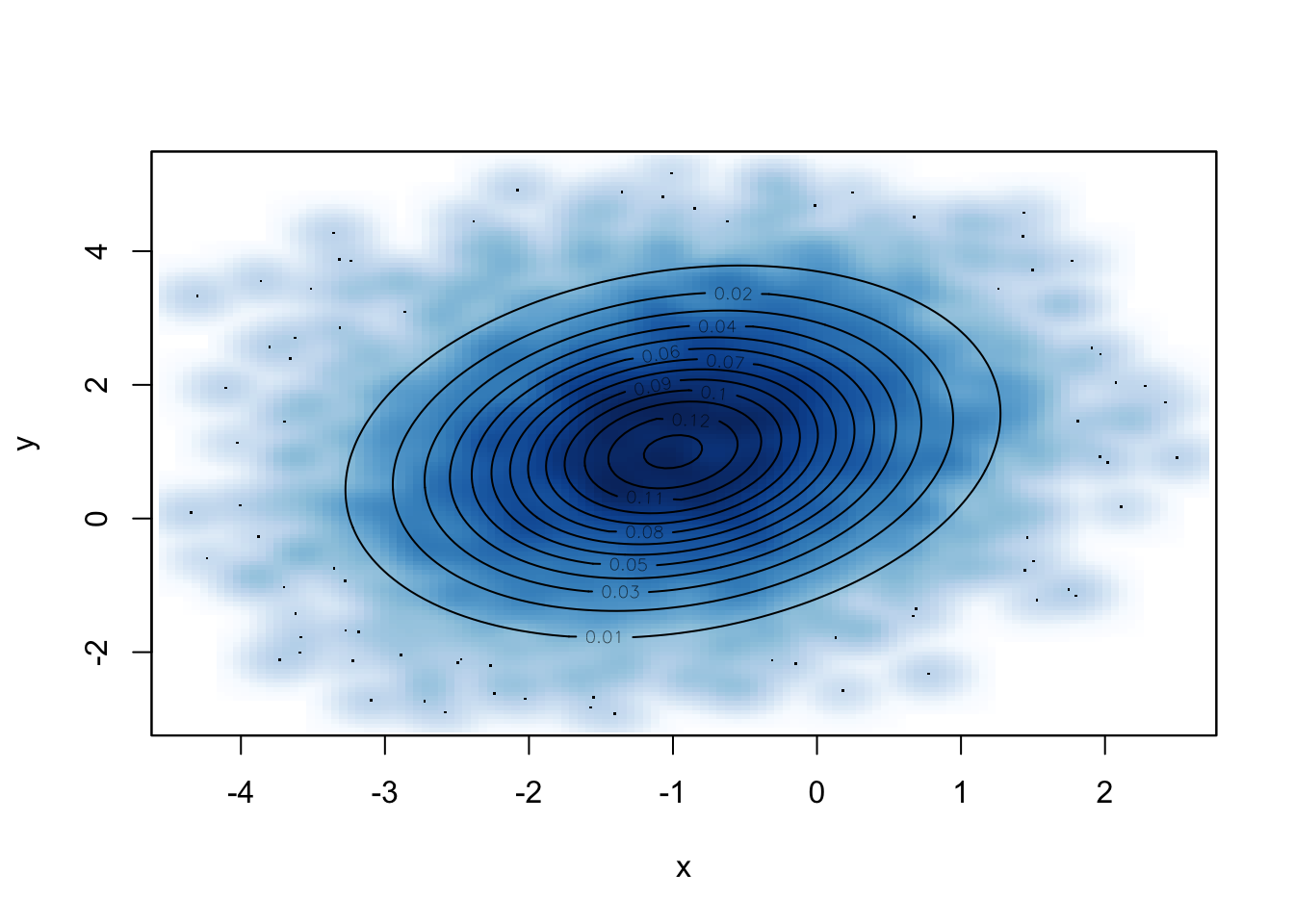### 8.3.2 独立 Metropolis-Hastings

• $$q$$ 中抽取 $$\epsilon$$，其中 $$q$$ 是一个以 0 为中心的对称分布。让 $$x^* = x_{t-1} + \epsilon$$

• 计算接受概率

$\alpha = \min\left(1, \frac{f(x^*)g(x_{t-1})}{f(x_{t-1})g(x^*)}\right).$

• 产生均匀分布的随机数 $$U \sim U(0,1)$$，如果 $$U \leq \alpha$$，接受 $$x^*$$，即以 $$\alpha$$ 为接受概率接受 $$x^*$$。若接受，$$x_t = x^*$$，否则 $$x_t = x_{t-1}$$

## 8.4 Gibbs 抽样

Gibbs 抽样算法

• $$f(x|y_i)$$ 中抽取 $$x_{i+1}$$

• $$f(y|x_{i+1})$$ 中抽取 $$y_{i+1}$$

1. $$f(x|y_i, z_i)$$ 中抽取 $$x_{i+1}$$

2. $$f(y|x_i, z_i)$$ 中抽取 $$y_{i+1}$$

3. $$f(z|x_i, y_i)$$ 中抽取 $$z_{i+1}$$

1. $$f(x|y_i) \sim N(\mu_1 + \rho \frac{\sigma_1}{\sigma_2}(y_i-\mu_2), \sigma_1^2(1-\rho))$$ 中抽取 $$x_{i+1}$$

2. $$f(y|x_{i+1}) \sim N(\mu_2 + \rho \frac{\sigma_2}{\sigma_1}(x_{i+1} -\mu_1), \sigma_2^2(1-\rho))$$ 中抽取 $$y_{i+1}$$

gibbs <- function(x0, mu, Sigma, n.steps = 1000){
mu1 <- mu
mu2 <- mu
sigma1 <- Sigma[1, 1]
sigma2 <- Sigma[2, 2]
rho <- Sigma[1, 2]
samples <- matrix(NA, n.steps, 2)
samples[1,1] <- x <- x0
samples[1,2] <- y <- x0

for (i in 1:n.steps-1) {
samples[i+1, 1] <- x <-
rnorm(1, mu1 +
rho * sigma1 * (y-mu2) /
sigma2, sqrt((sigma1^2) * (1 - rho^2) ))
samples[i+1, 2] <- y <-
rnorm(1, mu2 +
rho * sigma2 * (x-mu1) /
sigma1, sqrt((sigma2^2) * (1 - rho^2) ))
}
return(samples)
}
set.seed(Sys.Date())
mu = c(-1, 1)
Sigma = matrix(c(1, .25, .25, 1.5), 2, 2)
samples <- gibbs(c(-4,4), mu, Sigma, 10000)
smoothScatter(samples, xlab ='x', ylab = 'y')
contour(x, y, z, add = TRUE)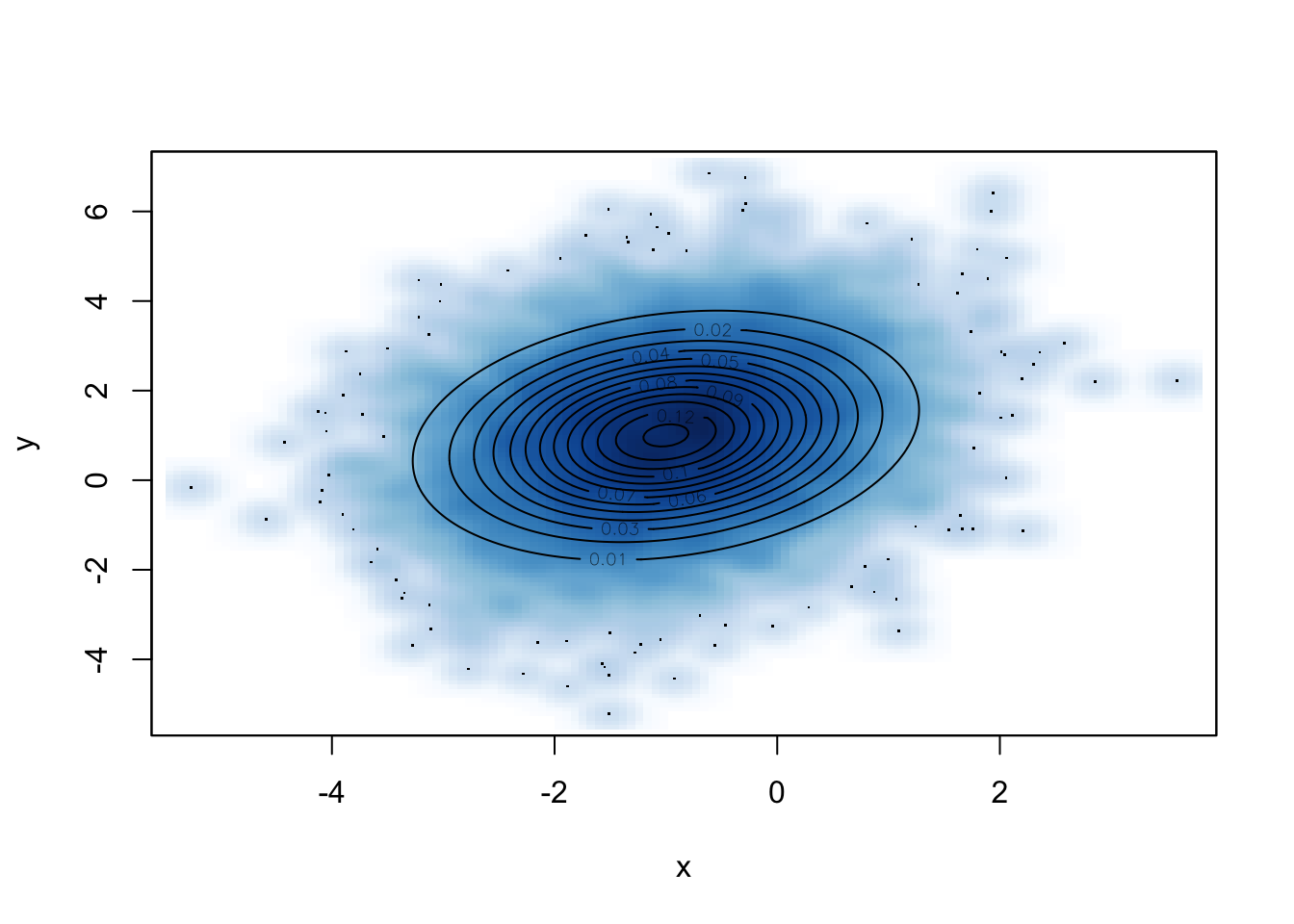## 8.6 MCMC 的收敛效率

# 定义不同的猜想概率
g1 <- function(x) rnorm(1, x, 0.1)
g2 <- function(x) rnorm(1, x, 4)
g3 <- function(x) rnorm(1, x, 50)

# 分别抽样
set.seed(Sys.Date())
samples1 <- rw.mh(-5, f, g1, n.steps)
samples2 <- rw.mh(-5, f, g2, n.steps)
samples3 <- rw.mh(-5, f, g3, n.steps)

# 画出路径图
samples <- data.frame(iter = 1:n.steps, samples1 = samples1,
samples2 = samples2, samples3 = samples3)
cggplot(samples, aes(iter)) +
geom_line(aes(y = samples1, colour = "sd = 0.1")) +
geom_line(aes(y = samples2, colour = "sd = 4")) +
geom_line(aes(y = samples3, colour = "sd = 50")) +
scale_colour_discrete(expression(sigma),
labels = c('0.1', '4', '50')) +
xlab('迭代次数') + ylab('抽样')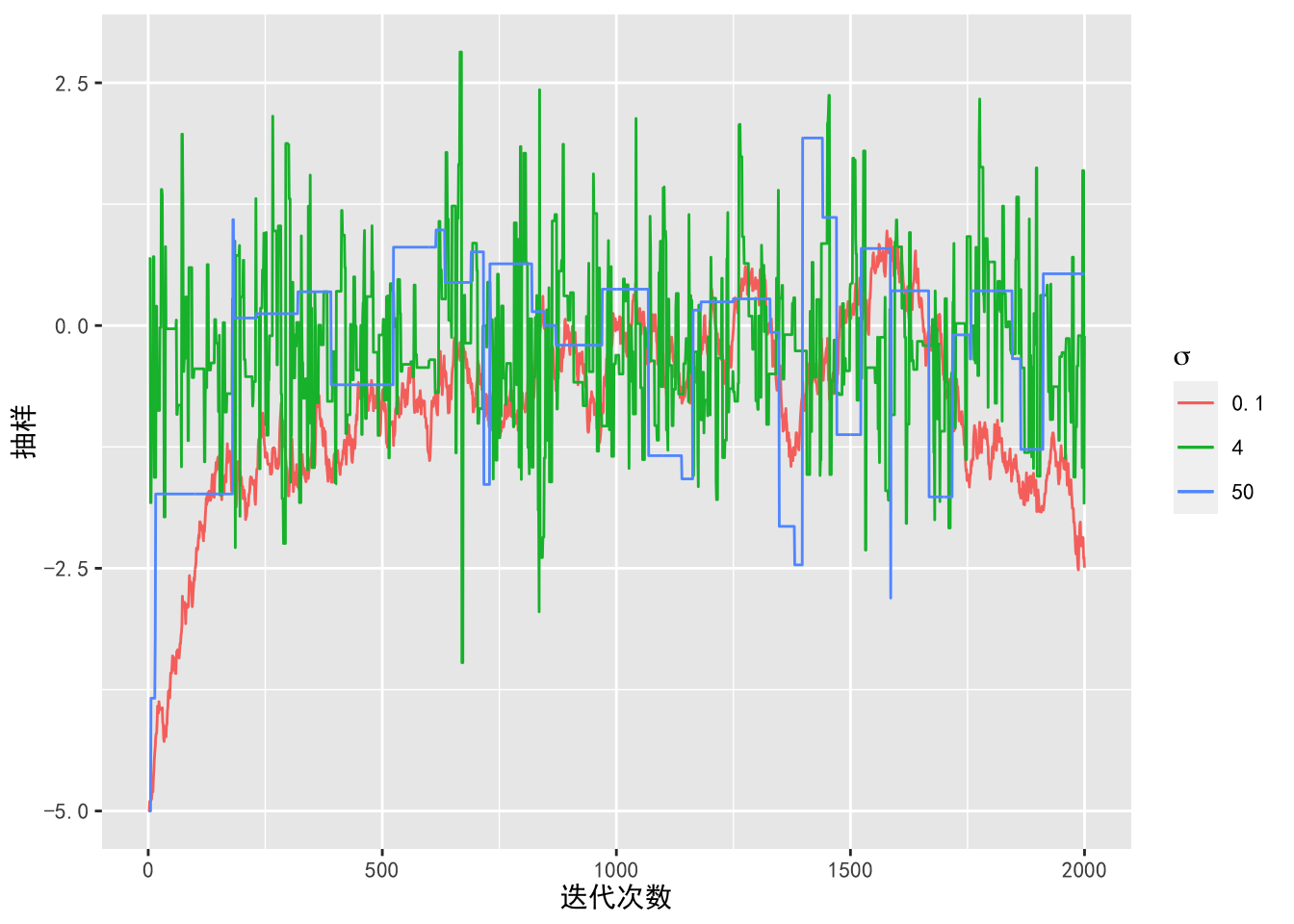library(reshape2)
library(dplyr)
samples.melt <- data.frame(iter = 1:n.steps,
samples1 = samples1,
samples2 = samples2,
samples3 = samples3) %>%
select(-iter) %>% melt()
cggplot(samples.melt,aes(x=value, color=variable)) +
geom_density(alpha=0.25, size = 1.3) +
scale_color_discrete(expression(sigma),
labels = c('0.1', '4', '50')) +
xlab('x') + ylab('密度')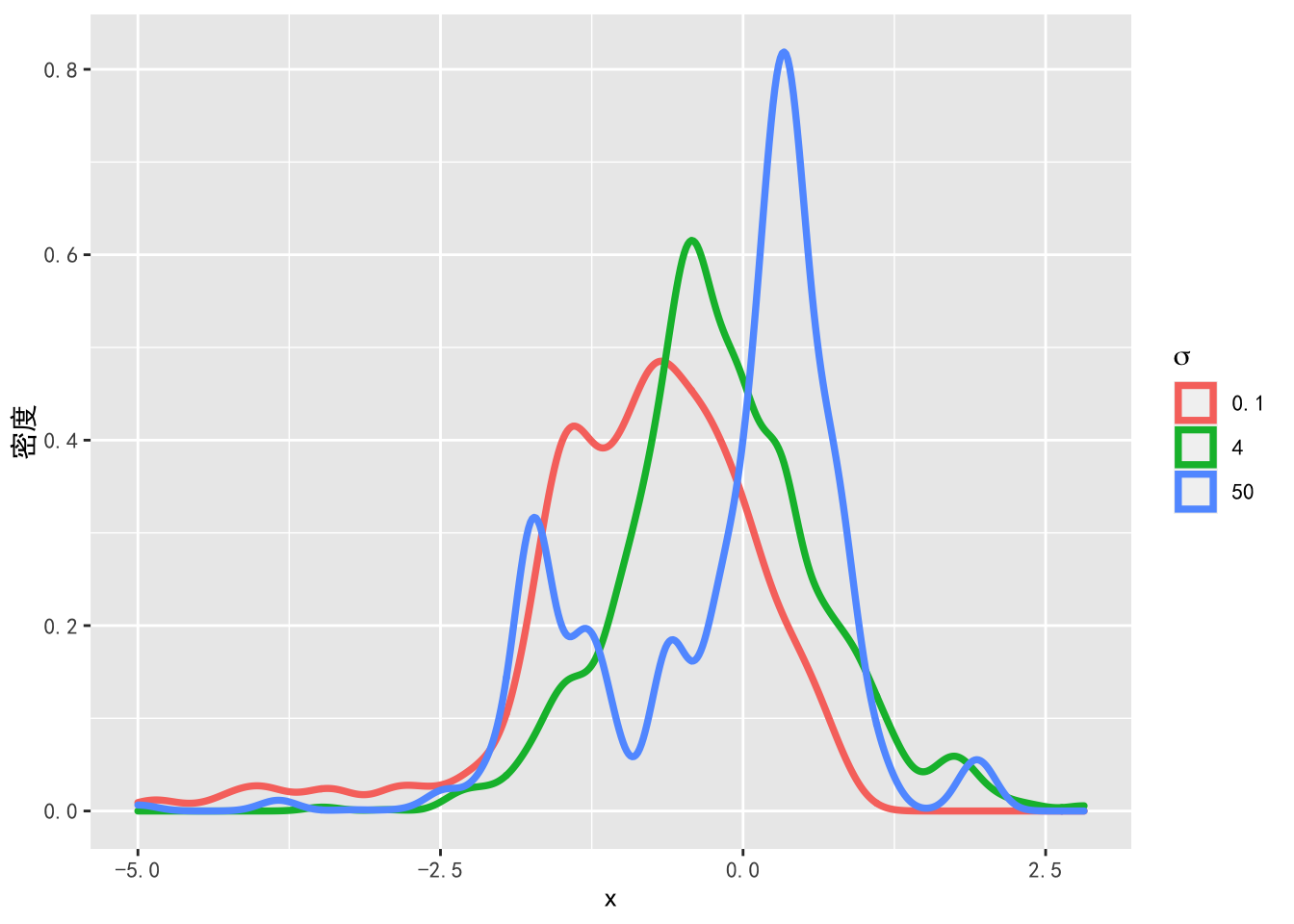### 8.6.1 蒙特卡洛误差

$E_X \approx \frac{1}{M}\sum\limits_{i = 1}^M x_i.$ 因为我们用样本均值进行估计 $$E_X$$，所以会导致估计误差，这个误差会随着抽样数量的增加而减小。我们把这个误差称作蒙特卡洛误差（Monte Carlo Error）。一种测量蒙特卡洛误差的方法是计算样本均值的方差，即

\begin{aligned} \operatorname{Var}\left(\frac{1}{M} \sum_{i=1}^{M} x_i\right) &=\frac{1}{M^{2}} \operatorname{Var}\left(\sum_{i=1}^{M} x_i\right) \\ &=\frac{1}{M^{2}} \sum_{i=1}^{M} \operatorname{Var}\left(x_i\right)+\frac{2}{M^{2}} \sum_{i=1}^{M} \sum_{j>i} \operatorname{cov}\left(x_i, x_j\right) \\ &=\frac{\operatorname{Var}(X)}{M}+\frac{2}{M^{2}} \sum_{i=1}^{M} \sum_{j>i} \operatorname{cov}\left(x_i, x_j\right) \end{aligned}. 由此可见，在马尔科夫链中样本之间相关性越小，样本均值的方差越小。 图8.5展示了例6.1中不同的猜想概率密度标准差下抽取样本的自相关图。当 $$\sigma = 4$$ 时，样本之间的自相关最小，这时蒙特卡洛误差最小。

ggcor <- function(x){
bacf <- acf(x, plot = FALSE)
bacfdf <- with(bacf, data.frame(lag, acf))
p <- ggplot(data = bacfdf,
mapping = aes(x = lag, y = acf)) +
geom_hline(aes(yintercept = 0)) +
geom_segment(mapping = aes(xend = lag, yend = 0))
return(p)
}

p1 <- ggcor(samples1) + ggtitle(bquote(sigma ~ "= 0.1"))
p2 <- ggcor(samples2) + ggtitle(bquote(sigma ~ "= 4"))
p3 <- ggcor(samples3) + ggtitle(bquote(sigma ~ "= 50"))
library(patchwork)
p1 / p2 / p3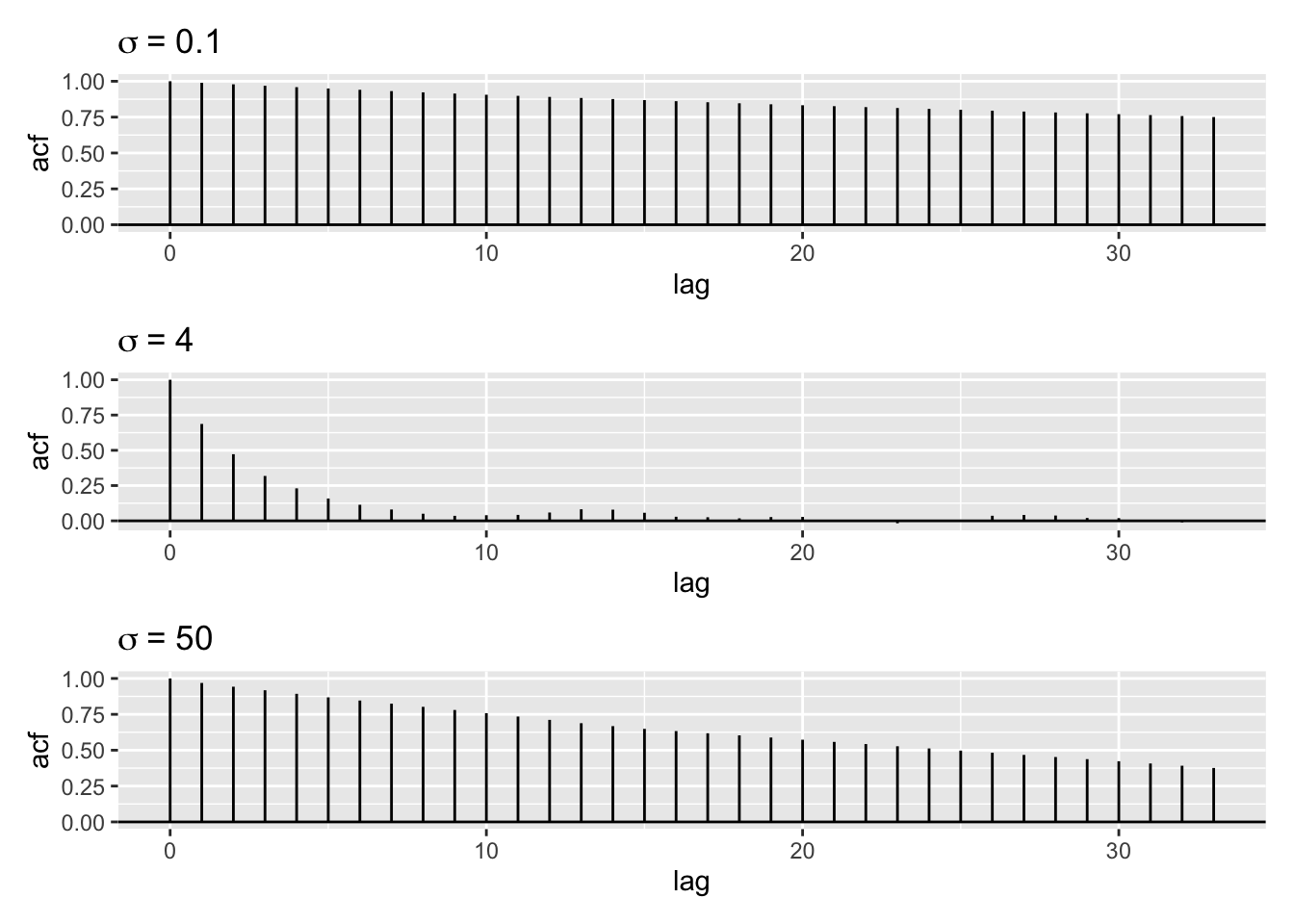### 8.6.2 有效样本量

$\operatorname{Var}\left(\frac{1}{M} \sum_{i=1}^{M} x_i\right) = \frac{\operatorname{Var}(X)}{M}+\frac{2}{M^{2}} \sum_{i=1}^{M} \sum_{j>i} \operatorname{cov}\left(x_i, x_j\right) = \frac{\operatorname{Var}(X)}{M_{\operatorname{ESS}}}.$

coda::effectiveSize(samples1)
#>  var1
#> 8.561
coda::effectiveSize(samples2)
#>  var1
#> 325.8
coda::effectiveSize(samples3)
#>  var1
#> 28.63

## 8.7 基于优化的抽样算法

1. 随机游走 Metropolis-Hastings 算法也就是最原始的 Metropolis 算法。↩︎